Download Presentation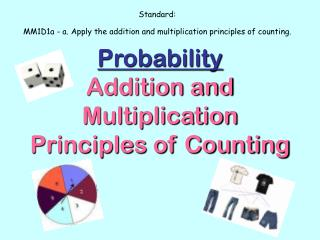Probability Addition and Multiplication Principles of Counting

# Probability Addition and Multiplication Principles of Counting - PowerPoint PPT Presentation

Probability Addition and Multiplication Principles of Counting. Standard: MM1D1a - a. Apply the addition and multiplication principles of counting. What is Probability?. The likelihood that a given event will occur. What real life situations would involve probability?.I am the owner, or an agent authorized to act on behalf of the owner, of the copyrighted work described.
Download Presentation## Probability Addition and Multiplication Principles of Counting

An Image/Link below is provided (as is) to download presentation

Download Policy: Content on the Website is provided to you AS IS for your information and personal use and may not be sold / licensed / shared on other websites without getting consent from its author.While downloading, if for some reason you are not able to download a presentation, the publisher may have deleted the file from their server.

- - - - - - - - - - - - - - - - - - - - - - - - - - E N D - - - - - - - - - - - - - - - - - - - - - - - - - -
Presentation Transcript### ProbabilityAddition and Multiplication Principles of Counting

Standard:

MM1D1a - a. Apply the addition and multiplication principles of counting.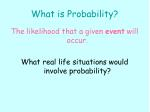What is Probability?

The likelihood that a given event will occur.

What real life situations would involve probability?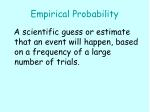Empirical Probability

A scientific guess or estimate that an event will happen, based on a frequency of a large number of trials.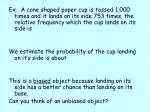Ex. A cone shaped paper cup is tossed 1,000 times and it lands on its side 753 times, the relative frequency which the cup lands on its side is

We estimate the probability of the cup landing on it’s side is about

This is a biased object because landing on its side has a better chance than landing on its base.

Can you think of an unbiased object?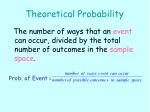Theoretical Probability

The number of ways that an event can occur, divided by the total number of outcomes in the sample space.

Prob. of Event =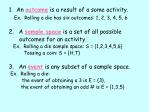1. An outcome is a result of a some activity.

Ex. Rolling a die has six outcomes: 1, 2, 3, 4, 5, 6

• A sample space is a set of all possible

outcomes for an activity.

Ex. Rolling a die sample space: S = {1,2,3,4,5,6}

Tossing a coin: S = {H,T}

• An event is any subset of a sample space.

Ex. Rolling a die:

the event of obtaining a 3 is E = {3},

the event of obtaining an odd # is E = {1,3,5}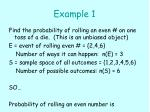Example 1

Find the probability of rolling an even # on one toss of a die. (This is an unbiased object)

E = event of rolling even # = {2,4,6}

Number of ways it can happen: n(E) = 3

S = sample space of all outcomes = {1,2,3,4,5,6}

Number of possible outcomes: n(S) = 6

SO…

Probability of rolling an even number is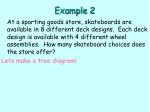Example 2

At a sporting goods store, skateboards are available in 8 different deck designs. Each deck design is available with 4 different wheel assemblies. How many skateboard choices does the store offer?

Lets make a tree diagram!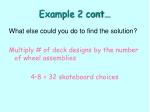Example 2 cont…

What else could you do to find the solution?

Multiply # of deck designs by the number of wheel assemblies

4•8 = 32 skateboard choices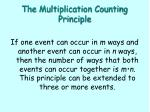The Multiplication Counting Principle

If one event can occur in m ways and another event can occur in n ways, then the number of ways that both events can occur together is m•n. This principle can be extended to three or more events.If the possibilities being counted can be divided into groups with no possibilities in common, then the total number of possibilities (outcomes) is the sum of the numbers of possibilities in each group.

HUH???Suppose that we want to buy a computer from one of two makes, Dell and Apple.

Suppose also that those makes have 12 and 18 different models, respectively. Then how many models are there altogether to choose from ?Solution

Since we can choose one of 12 models of Dells or one of 18 of Apples, there are all together 12 + 18 = 30 models to choose from.

This is the Addition Principle of Counting. Choosing one from given models of either make is called an event and the choices for either event are called the outcomes of the event. Thus the event "selecting one from make Dell", for example, has 12 outcomes.What’s the Probability?

How would we find the probability of choosing a Dell?

12 Dells = 2

30 total choices 5

The probability of choosing a Dell would be:

2 = .4 = 40%

5Example 3

Codes- Every purchase made on a company’s website is given a randomly generated confirmation code. The code consists of 3 symbols (letters and digits). How many codes can be generated if at least one letter is used in each?Example 3 - Solution

To find the number of codes, find the sum of the numbers of possibilities for 1-letter codes, 2-letter codes and 3-letter codes.

1-letter: 26 choices for each letter and 10 choices for each digit. So 26•10•10 = 2600 letter-digit-digit possibilities. The letter can be in any of the three positions , so there are 3•2600 = 7800 total possibilities.Example 3 - Solution

2-letter: There are 26•26•10 = 6760 letter-letter-digit possibilities. The letter can be in any of the three positions , so there are 3•6760 = 20,280 total possibilities.

3-letter: There are 26•26•26 = 17,576 possibilities

Total Possibilities: 7800 + 20,280 + 17,576 = 45, 656 possible codes